Next: Magnetic Induction Up: Magnetism Previous: Example 8.2: Charged particle

## Example 8.3: Ampère's circuital law

Question: A-directed wire of radiuscarries a total-directed current. What is the magnetic field distribution, both inside and outside the wire, if the current is evenly distributed throughout the wire? What is the magnetic field distribution if the current is concentrated in a thin layer at the surface of the wire?

Answer: Since the current distribution possesses cylindrical symmetry, it is reasonable to suppose that the magnetic field it generates also possesses cylindrical symmetry. By analogy with the magnetic field generated by an infinitely thin-directed wire, we expect the magnetic field to circulate in the-plane in an anti-clockwise direction (looking against the direction of the current). Let us apply Ampère's circuital law to a circular loop in the-plane which is centred on the centre of the wire, and is of radius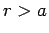. The magnetic field is everywhere tangential to the loop, so the line integral of the magnetic field (taken in an anti-clockwise sense, looking against the direction of the current) is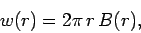where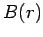is the magnetic field-strength at radius. According to Ampère's circuital law, this line integral is equal to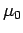times the total current enclosed by the loop. The total current is clearly, since the loop lies outside the wire. Thus,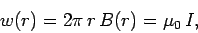giving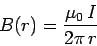for. This is exactly the same field distribution as that generated by an infinitely thin wire carrying the current. Thus, we conclude that the magnetic field generated outside a cylindrically symmetric-directed current distribution is the same as if all of the current were concentrated at the centre of the distribution. Let us now apply Ampère's circuital law to a circular loop which is of radius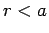. The line integral of the magnetic field around this loop is simply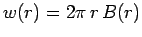. However, the current enclosed by the loop is equal totimes the ratio of the area of the loop to the cross-sectional area of the wire (since the current is evenly distributed throughout the wire). Thus, Ampère's law yields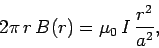which gives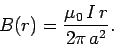Clearly, the field inside the wire increases linearly with increasing distance from the centre of the wire.

If the current flows along the outside of the wire then the magnetic field distribution exterior to the wire is exactly the same as that described above. However, there is no field inside the wire. This follows immediately from Ampère's circuital law because the current enclosed by a circular loop whose radius is less than the radius of the wire is clearly zero.Next: Magnetic Induction Up: Magnetism Previous: Example 8.2: Charged particle
Richard Fitzpatrick 2007-07-14